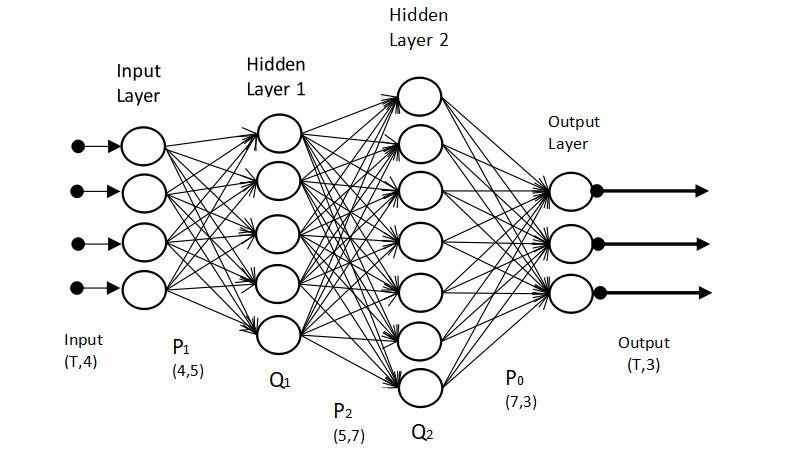Artificial Intelligence A device takes in information, does some processing to complete the task successfully.

A system that perceives information from the environment understands and interprets the data to take required action is known as Artificial intelligence machines.

A system that maximizes its chance of success by properly analyzing data is the core of Artificial intelligence. To develop Artificial intelligence (AI) products hardcoded instructions or program is of no use as the data around us is huge.

So as to develop generic solution algorithms are designed in such a way that enables machines to learn the required pattern, gain intelligence over time to take make a good decision.

How do humans learn?

Acquiring new things and updating our skills, knowledge is the process of learning. God blessed humans and every creature on the planet with this skill. With experience, we learn and grow.

Due to continuous interaction with society and the environment consciously and unconsciously we all are learning.

Five traditionally recognized senses of humans are sight (eyes), smell (nose), touch (skin), hearing, taste (tongue). All the species in the world have a multitude of senses.

With these senses we continuously gather information, neurons carry information our brain interprets the signals, processes, integrates and coordinates to take decisions.

How AI mimics biological Neurons?

The brain's basic working unit is neurons. The human brain is made up of 100 billion neurons. These neurons transmit information to and from the brain and to the various parts of the body.

Biological Neurons: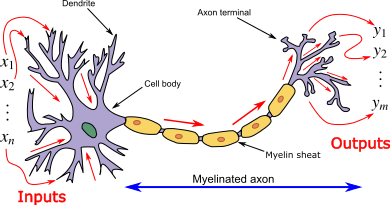Deep Learning is a subset of Artificial Intelligence that consists of algorithms inspired by the function and structure of the brain.

Artificial neurons are inspired by biological neurons. The neurons are connected to each other to control body functions, emotions, and movements.

The key components of biological neurons are: Dendrites bring information to soma so dendrites accept stimuli from an external environment.

Soma is the spherical part of neurons, the incoming signals are summed up by the soma when sufficient input is received neurons fire up(i.e. when the threshold is exceeded) and the axon sends information to other neurons depending upon the strength of the signal. If the input is not sufficient no potential action is taken.

Artificial Neuron: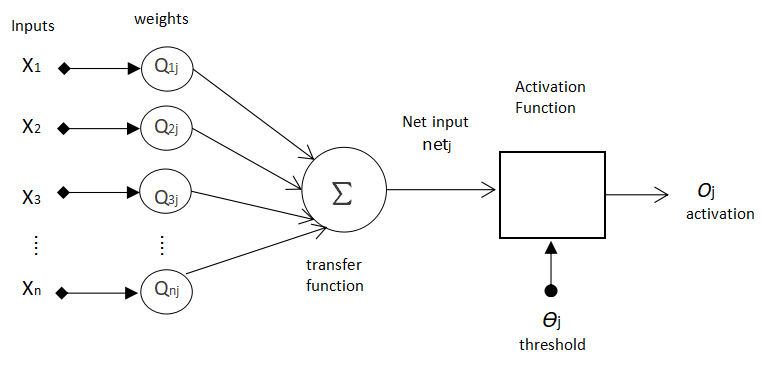An elementary unit in Artificial Neural Network is Artificial Neuron. The artificial neuron is primarily composed of Inputs, weights, Activation function and Output. Each input has an associated weight (w). The input is summed up and a non-linear activation function is applied to it. The output is given at the output line.

Working of Artificial Neural Network :

1. Multiply inputs by its weight. For example : x1.w1j
2. Calculate the weighted sum for each input and weight. ?wj
3. The netput is given to the activation function to determine the output. If the weighted sum is greater than the threshold value assigns 1 and else 0 as output.

Artificial Neural Network (ANN):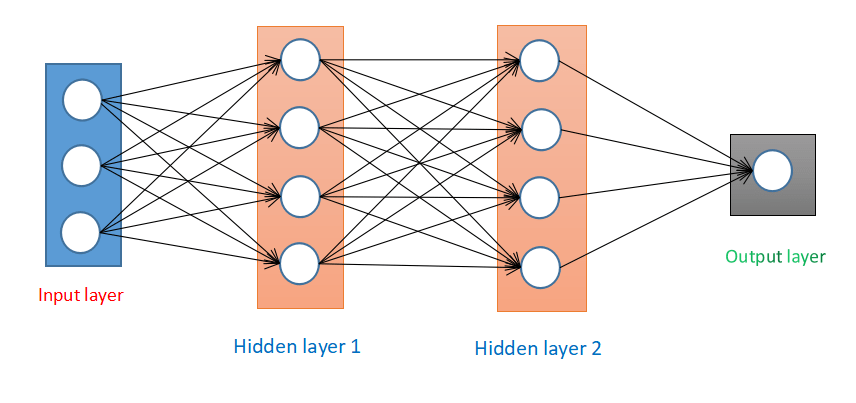Artificial Neural network is composed of multiple nodes which take input process them and give output. Each node output is known as activation or node value.

ANN consists of the input layers, hidden layers and output layers. At the hidden layer, input is transformed to derive some pattern that can be given at the output layer.

Types of Neural Network in Artificial Intelligence:

The Different Types of Neural network are:

1. Convolutional Neural Network
2. Feed Forward Neural Network
3. Radial basis Function Neural Network
4. Multilayer Perceptron
5. Recurrent Neural Network
6. Long short-term memory
7. Long short-term memory
8. Input Layer
9. Convolutional Layers
10. Pooling Layers
11. Fully connected Layers
• Convolutional Neural Network (CNN)

Convolutional Neural Network works best for Images and videos which is a class of Deep Neural Networks. It has input layers, output layers and multiple hidden layers which are series of convolutional layers.

The building blocks are

1. Input Layer
2. Convolutional Layers
3. Pooling Layers
4. Fully connected Layers

As we can see in the diagram first input image with its height and weight parameter is feed at the input layer. The image is convoluted and passed to the next layer. Pooling layers is generally use to manage the dimensions of the data. Finally, the output is flattened and given at the output layer.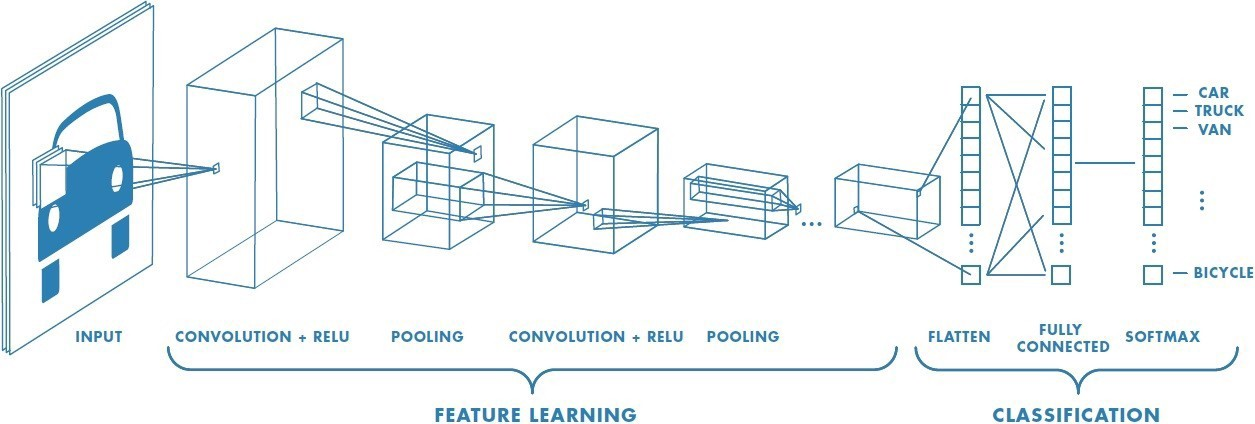• Feed Forward Neural Network

It is one of the simplest forms of the network where data (input) travels in the only direction. In this network, there is no backpropagation, and connections between nodes do not form a cycle or loops. Perception is the simplest feed-forward neural network.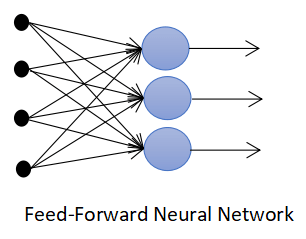• Recurrent Neural Network (RNN)It is a network where the flow of the data is not restricted to one direction. It has more capability and greater learning speed which is used to solve complex tasks.  In this network output from the next step is feedback to the previous state. RNN has a memory that retains information for processing

• Bayesian Network:

The draw a probabilistic relationship between a set of the variables Bayesian network is used.

They are used for a wide variety of tasks such as decision making, prediction, anomaly detection etc.

It is a cyclic graph that denotes both random variables and conditional dependencies.

• Modular Neural Network:

It is a collection of neural networks working independently where each neural network has a set of inputs.

The advantage of a Modular Neural network is that it divides the large computational task into smaller modules and thus decreases the complexity.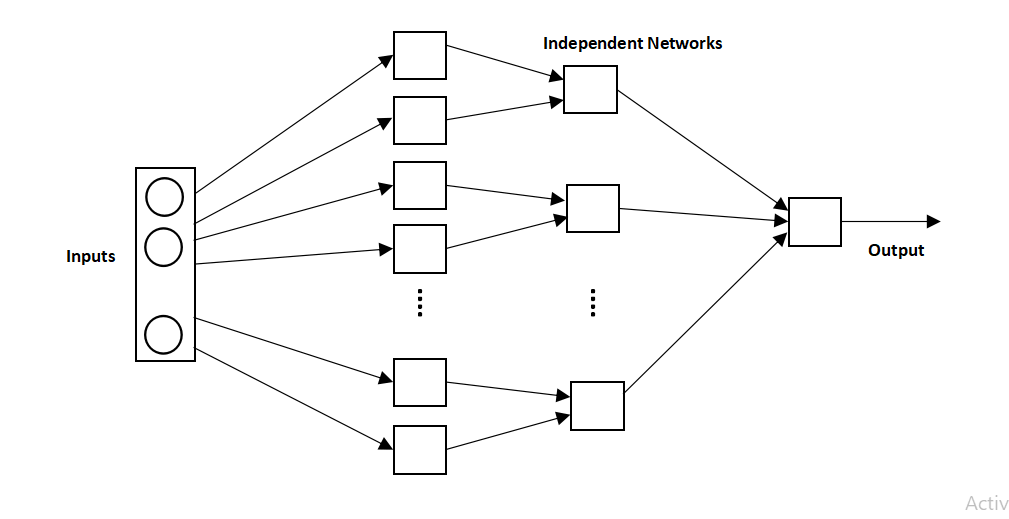Applications of Artificial Neural Network:

• Handwriting Recognition
• Face Detection and Recognition: In the upcoming days face Recognition will be a popular biometric. To extract features from millions of faces is a big task that can be accomplished by an Artificial neural network.
• Image Compression: In this era, data is generated every second. Most of the applications and sites are using images either data transfer takes place or images are loaded on the websites so using a neural network to reduce the size of the image is worth it.
• Speech Recognition: To eliminate the communication barrier between humans and computers sophisticated neural networks can be made to understand the spoken language of humans. Attempts to make speech recognition systems are made using Multi-layer networks, Kohonen self-organizing maps etc.

Learn Deep Learning and Artificial Intelligence

In a similar way, numerous applications can be made using an Artificial Neural network.

The artificial Neural Network model is very good for problem-solving which is flexible and powerful. Without doing any hard-coded instruction just by training network with examples we can reap good results.

Deep Learning is the subset of Artificial Intelligence and it is a blooming field of this decade.